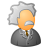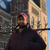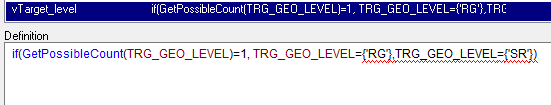# QlikView App Dev

Discussion Board for collaboration related to QlikView App Development.

cancel
Showing results for
Did you mean:Creator

## Variable in Set Analysis

Hi experts!

I am trying to define a variable that will be used in several expressions. This variable has a set analysis in it which also has a variable inside. It is like this:

vTarget_level = if(GetPossibleCount(TRG_GEO_LEVEL)=1, TRG_GEO_LEVEL={'RG'},TRG_GEO_LEVEL={'SR'});

vTargetCSAT_TablChart= sum({<TRG_CODE={'CSAT'}, \$(=vTarget_level)>} TRG_TARGET * TRG_Target_Weights)/sum({<TRG_CODE={'CSAT'},\$(=vTarget_level)>}TRG_Target_Weights)

I use it on a text object as:

=\$(vTargetCSAT_TablChart)

What gives error. If i put in the text box:

=(vTargetCSAT_TablChart)

Results: sum({<TRG_CODE={'CSAT'},>} TRG_TARGET * TRG_Target_Weights)/sum({<TRG_CODE={'CSAT'},>}TRG_Target_Weights)

How should I do it so it gives the result?? Thanks.4 RepliesChampion

Hi,

verify that you didn't put an '=' when declaring both variables.

and use this:

=\$(vTargetCSAT_TablChart)Creator
Author

There is no '=' in the declaration. They are both like thisChampion

Actually i didn't read the content of the variables and the association between them.

did you try your first variable before ? the output should be a stringMaster II

try this

vTarget_level=  if(GetPossibleCount(TRG_GEO_LEVEL)=1, 'TRG_GEO_LEVEL={'RG'}','TRG_GEO_LEVEL={'SR'}');

not put it in a text box and check

Learning never stops.